## Given problem

Suppose we have an array, we need to sort this array followed by increasing order.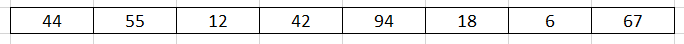In this article, we will use insertion sort to deal with this problem.

## How insertion sort works

The idea of insertion sort is that assuming that based on an sorted array `a, ..., a[i - 1]`, we want to determine a suitable position of a[i] element to insert it into this array.

Normally, we will go backward from `i - 1` index to 0 index. If `a[i] < a[i - 1]`, swap them.

Belows are some steps to use insertion sort with the above array.

1. Original array2. Insert a new element at the index = 1 into the array’s length = 1, from index = 0.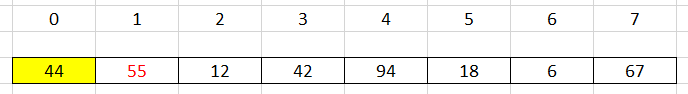We can find that a new element with value = 55 is always greater than 44. So, we do not swap them.

Then, our new array that contains both 44, 55 is sorted.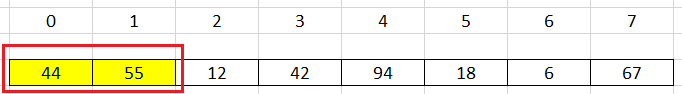3. Insert a new element at the index = 2 into the array’s length = 2, from index = 0.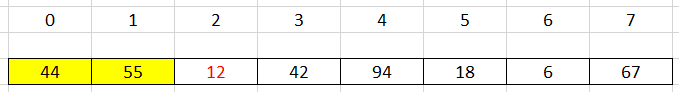A new element has value = 12 that is less than other elements in our new array.

Applying the idea of insertion sort, go backward from index = 2 to index = 0, compare a couple element, then swap them.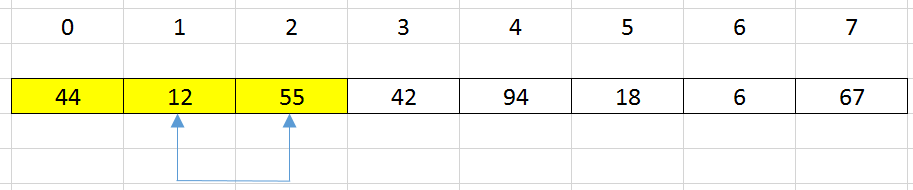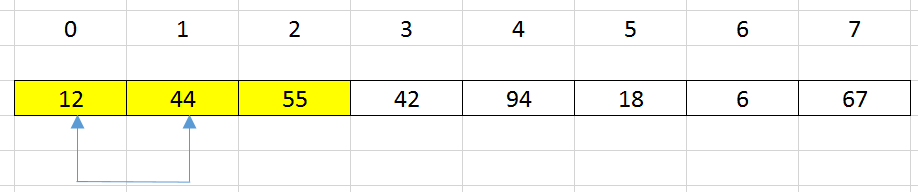Now, we have our new sorted array: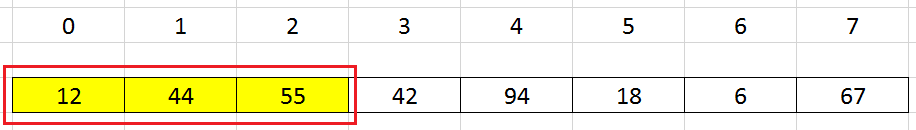4. Insert a new element at the index = 3 into the array’s length = 3, from index = 0.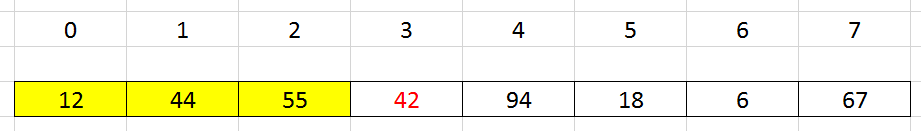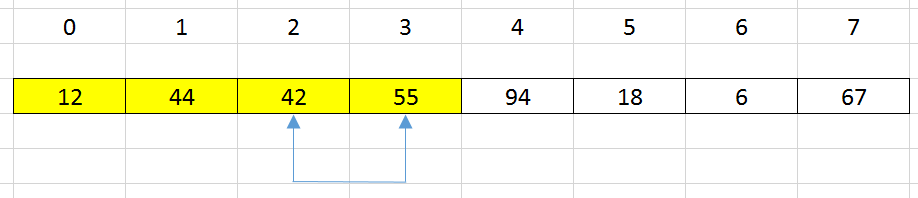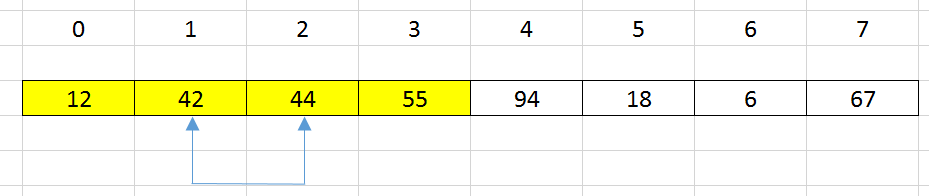Then, we have a sorted array: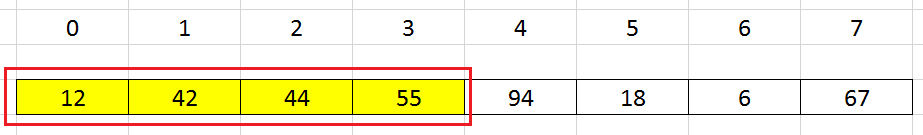5. Continue to the other elements, we have the original sorted array.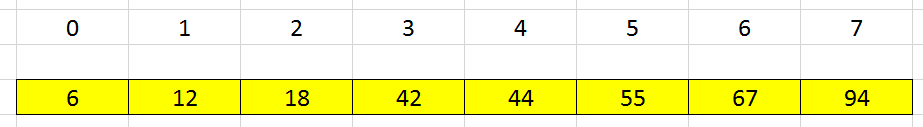## Source code

Belows are some versions of the insertion sort’s implementation.

1. Version 1

`````` public static void insertionSort(int[] nums) {
int length = nums.length;

for (int i = 1; i < length; ++i) {
for (int j = i; j > 0; --j) {
if (nums[j] < nums[j - 1]) {
// exchange value between nums[j] and nums[j - 1]
int tmp = nums[j];
nums[j] = nums[j - 1];
nums[j - 1] = tmp;
}
}
}
}

``````
2. Version 2

`````` public static void insertionSortVersion2(int[] nums) {
int length = nums.length;

for (int i = 1; i < length; ++i) {
int key = nums[i];
int j = i - 1;

// shift elements to right side if key < nums[j]
while (j >= 0 && key < nums[j]) {
nums[j + 1] = nums[j];
--j;
}

nums[j + 1] = key;
}
}

``````

The complexity of the insertion sort is:

• Time complexity:

• Worst case: O(n^2) with an array in the descending order.
• Space complexity: O(1)

Insertion sort is a stable sorting algorithm because the order of equal elements is maintained after sorted.

## When to use

• When our array is nearly sorted.

• When our array has the small size.

• When we need to sort elements online - that is sorting them as they come in.

Because the idea of insertion sort is that suppose we have an sorted array, then insert a new element into it. Our new array is still sorted.

## Wrapping up

• Understanding about some versions of insertion sort: exchange and shift elements.

Refer:

https://www.acodersjourney.com/insertion-sort/